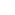Part of the Oxford Instruments Group
Expand

# Bounding Box Statistical VariableThis tutorial is about the bounding box statistical variable. A bounding box is a rectangular cuboid in 3D, or a rectangle in 2D, containing a single detected object. With this statistical variable, you can easily determine the size of any created objects.

The size of an object is an important characteristic and it is one of the basic ones used to describe an object. In Imaris, you can use the Measurement Points feature and determine any object dimension. However, accurately and precisely setting measurement points could be challenging and time consuming, especially for complex shaped objects. With the bounding box statistical variables the efficiency and accuracy could be improved, making even the measurement of the most complex objects easy.

The bounding box statistical variables will be shown using the confocal 3D Drosophila embryos image. This images are provided by Dominique Förster and Dr. Stefan Luschnig, the Institute of Molecular Life Sciences, University of Zurich.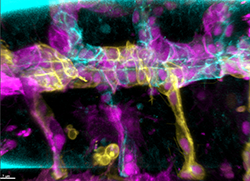In their study, Imaris Contour Drawing Mode was used to quantify cell surfaces and to calculate cell elongation and orientation within the tube epithelium.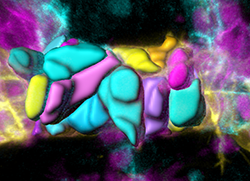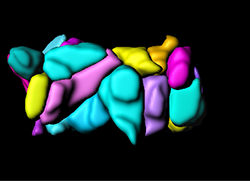To measure the longest diagonal inside each cell is the Measurement Points feature was used.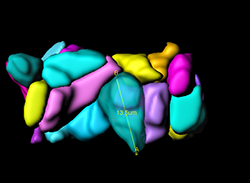Alternatively, under the Statistics Tab, the automatically calculated bounding box statistics are shown. Imaris offers two types of Bounding Box calculations: axis-aligned noted as Bounding Box AA and object-oriented noted as Bounding Box OO.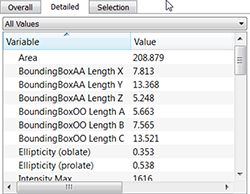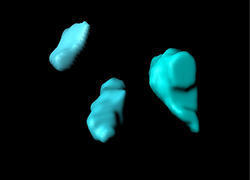If the bounding box is aligned with the axes of the coordinate system, it is known as an axis-aligned bounding box. The axis-aligned Bounding Box identifies an object by fully enclosing it within the minimal rectangular box which the object, whose local axes are aligned parallel to the coordinate system. The actual dimensions of the Bounding Box AA are based on the maximum and minimum position values of the along each of the X, Y and Z axes.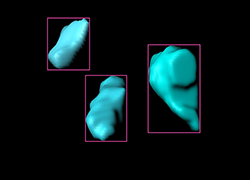The object-oriented Bounding Box identifies an object by fully enclosing it within the minimal rectangular box, whose local axes are aligned the same way as the principal axes of the object. The principal axes determine the orientation of the object and position of the object-oriented Bounding Box. The yellow arrows show the principal axes of the object.

The object-oriented Bounding Box is arbitrarily oriented with respect to the coordinate system. The furthest points with respect to the principal axes are used to determine the dimensions of the object-oriented bounding box.

BoundingBoxOO Length A measure the length of the shortest principal axis, BoundingBoxOO Length B measure the length of the second longest principal axis and BoundingBoxOO Length C measure the length of the longest principal axis inside of the object.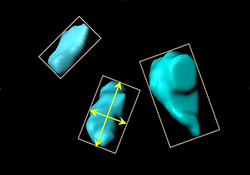For the selected objects, the axis-aligned bounding boxes are shown in magenta, while the object-oriented bounding box is shown in light color.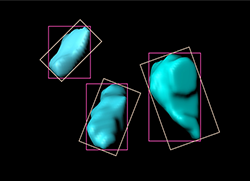The full Case Study related to the data used for this video tutorial can be found in our learning center, under the title Live Imaging Analysis Reveals Mechanisms Controlling Tube Size During Organ Development.

Category: Tutorial